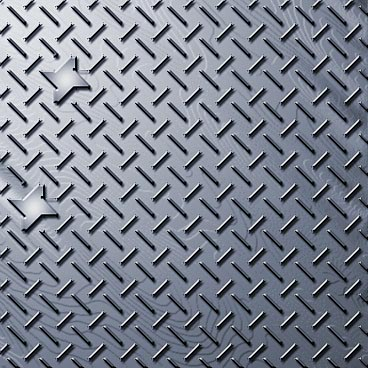### Creating a Metal Grate

This is a bit of a new experiment for me. After writing over 50 tutorials and spending over 5 years as a Technical Writer, I thought I would try a new approach by providing a pattern recipe as opposed to a tutorial. It may look daunting at first, but don't shy away. It's really quite simple once you try it out. And I promise I'll give you a smattering of screenshots along the way for some of those hard-to-handle dialogs. So take it slow and follow along to create a Metal Grate using nothing but Photoshop as your guide. And please let me know if you like this style of tutorial by going to my Forum and letting me know. If it's something you want to see more of, then I'd be happy to put a few other ones together.

So let's get right into it shall we?

Create a New Document with the following settings (your size can be variable):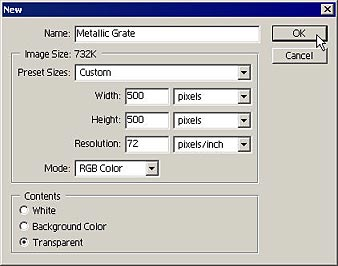Reset Swatches to default

Filter -> Render -> Clouds

Filter -> Render -> Difference Clouds (x6)

Filter -> Pixelate -> Mosaic

Cell Size = 40 square

Filter -> Stylize -> Find Edges

Channel = Composite Channel

Input = 155, 1.00, 255

Filter -> Brush Strokes -> Dark Strokes

Balance = 10

Black Intensity = 10

White Intensity = 0

Filter -> Brush Strokes -> Accented Edges

Edge Width = 11

Edge Brightness = 0

Smoothness = 1

Filter -> Brush Strokes -> Angled Strokes

Direction Balance = 100

Stroke Length = 40

Sharpness = 10

Select -> Color Range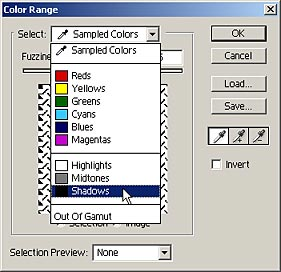Layer -> New -> Layer via Cut

Move the current layer (Cut Layer) to the bottom layer.

Filter -> Render -> Clouds

Point (Input = 0 Output = 0)

Point (Input = 16 Output = 249)

Point (Input = 52 Output = 10)

Point (Input = 71 Output = 249)

Point (Input = 102 Output = 12)

Point (Input = 126 Output = 230)

Point (Input = 155 Output = 9)

Point (Input = 174 Output = 249)

Point (Input = 203 Output = 3)

Point (Input = 220 Output = 239)

Point (Input = 255 Output = 255)

* Save this Curve, as you will be using it after the next step — See below.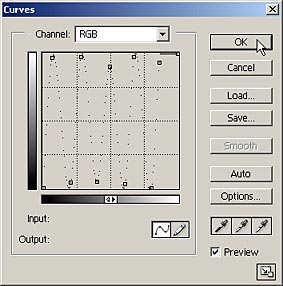Filter -> Blur -> Motion Blur

Angle = 42 degrees

Distance = 58 pixels

Image -> Adjustments -> Curves (enter the same points as before, or, if you previously saved the curve, Load this curve now, and apply it).

Filter -> Blur -> Gaussian Blur

Filter -> Blur -> Motion Blur

Angle = 42 degrees

Distance = 58 pixels

Select -> All

Edit -> Copy (Ctrl+C)

Select -> Save Selection. Click OK.

Go to the Channels palette and highlight the Alpha 1 channel

Edit -> Paste (Ctrl+V)

Select -> Deselect

Still within the Channels palette, highlight the RGB Channel.

Edit -> Fill

Use = 50% Gray

Mode = Normal

Opacity = 100%

Still within the Channels palette, highlight the Alpha 1 channel.

Filter -> Blur -> Motion Blur (x2)

Angle = -45 degrees

Distance = 198 pixels

Filter -> Blur -> Gaussian Blur

Still within the Channels palette, highlight the RGB Channel again.

Filter -> Render -> Lighting Effects

Light Type = Spotlight

Intensity = 35

Focus = 100

Gloss = 100

Material = 100

Exposure = -22

Ambience = -22

Texture Channel = Alpha 1

Uncheck the "White is High" checkbox

Height = 100Select Layer 1

Layer -> Layer Style -> Drop Shadow

Change Distance to 3, Size to 3, and Angle to 120 degrees (global)

Layer -> Layer Style -> Inner Shadow

Change Distance to 1, and Size to 25

Layer -> Layer Style -> Bevel and Emboss

Style = Inner Bevel, Technique = Chisel Hard, Depth = 1000, Size = 1 Highlight Mode = Screen; Opacity = 72 Shadow Mode = Multiply; Opacity = 100

Select -> All

Select -> Transform Selection (in the Options bar, click the bottom–right corner on the "Reference Point Location" grid)

Width = 92%, Height = 92%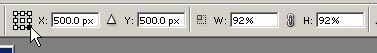The following shows the area that the selection should encompass. The idea is to delete the outer edges on the top and left of the image, as they are incongruous to the rest of the pattern.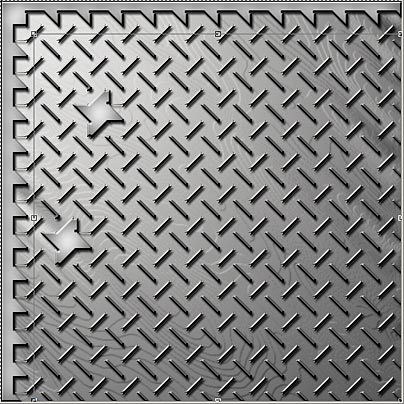When you have finished transforming the selection, press Enter/Return, or click on the Green arrow on the Options bar. This accepts the transformation.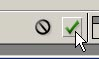Image -> Crop

Select -> Deselect

Layer -> Flatten Image